Test: Series-Series Connection of Two Port Network

# Test: Series-Series Connection of Two Port Network

Test Description

## 15 Questions MCQ Test Network Theory (Electric Circuits) | Test: Series-Series Connection of Two Port Network

Test: Series-Series Connection of Two Port Network for Electrical Engineering (EE) 2023 is part of Network Theory (Electric Circuits) preparation. The Test: Series-Series Connection of Two Port Network questions and answers have been prepared according to the Electrical Engineering (EE) exam syllabus.The Test: Series-Series Connection of Two Port Network MCQs are made for Electrical Engineering (EE) 2023 Exam. Find important definitions, questions, notes, meanings, examples, exercises, MCQs and online tests for Test: Series-Series Connection of Two Port Network below.
Solutions of Test: Series-Series Connection of Two Port Network questions in English are available as part of our Network Theory (Electric Circuits) for Electrical Engineering (EE) & Test: Series-Series Connection of Two Port Network solutions in Hindi for Network Theory (Electric Circuits) course. Download more important topics, notes, lectures and mock test series for Electrical Engineering (EE) Exam by signing up for free. Attempt Test: Series-Series Connection of Two Port Network | 15 questions in 45 minutes | Mock test for Electrical Engineering (EE) preparation | Free important questions MCQ to study Network Theory (Electric Circuits) for Electrical Engineering (EE) Exam | Download free PDF with solutions
 1 Crore+ students have signed up on EduRev. Have you?
Test: Series-Series Connection of Two Port Network - Question 1

### In the figure given below, the pole-zero plot corresponds to _____________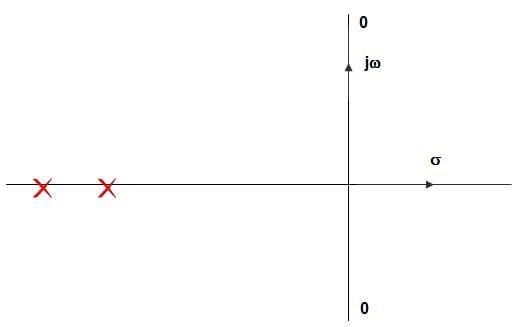Detailed Solution for Test: Series-Series Connection of Two Port Network - Question 1

In pole zero plot the two transmission zeroes are located on the jω-axis, at the complex conjugate location, and then the magnitude response exhibits a zero transmission at ω – ωC.

Test: Series-Series Connection of Two Port Network - Question 2

### A moving coil of a meter has 250 turns and a length and depth of 40 mm and 30 mm respectively. It is positioned in a uniform radial flux density of 450 mT. The coil carries a current of 160 mA. The torque on the coil is?

Detailed Solution for Test: Series-Series Connection of Two Port Network - Question 2

Given, N = 250, L = 40 x 10-3, d = 30 x 10-3m, I = 160 x 10-3A, B = 450 x 10-3 T
Torque = 250 x 450 x 10-3 x 40 x 10-3 × 30 x 10-3 x 160 x 10-3
= 200 x 10-6 N-m = 0.0216 N-m.

Test: Series-Series Connection of Two Port Network - Question 3

### In the circuit given below, the 60 V source absorbs power. Then the value of the current source is ____________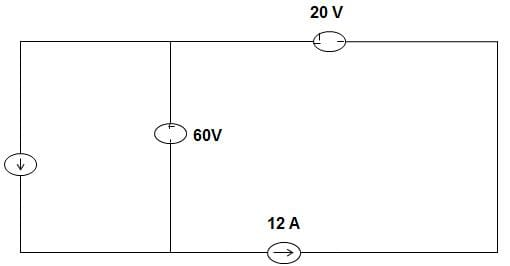Detailed Solution for Test: Series-Series Connection of Two Port Network - Question 3

Given that, 60 V source is absorbing power, it means that current flow from positive to negative terminal in 60 V source.
Applying KVL, we get, I + I1 = 12 A …… (1)
Current source must have the value of less than 12 A to satisfy equation (1).

Test: Series-Series Connection of Two Port Network - Question 4

For a 2 port network, the transmission parameters are given as 10, 9, 11 and 10 corresponds to A, B, C and D. The correct statement among the following is?

Detailed Solution for Test: Series-Series Connection of Two Port Network - Question 4

Here, A = 10, B = 9, C = 11, D = 10
∴ A = D
∴ Condition for symmetry is satisfied.
Also, AD – BC = (10) (10) – (9) (11)
= 100 – 99 = 1
Therefore the condition of reciprocity is satisfied.

Test: Series-Series Connection of Two Port Network - Question 5

The condition for a 2port network to be reciprocal is ______________

Detailed Solution for Test: Series-Series Connection of Two Port Network - Question 5

If the network is reciprocal, then the ratio of the response transform to the excitation transform would not vary after interchanging the position of the excitation.

Test: Series-Series Connection of Two Port Network - Question 6

How many incandescent lamps connected in series would consume the same total power as a single 100 W/220 V incandescent lamp. The rating of each lamp is 200 W/220 V?

Detailed Solution for Test: Series-Series Connection of Two Port Network - Question 6

In series power  = 1/p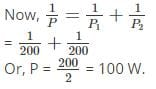Test: Series-Series Connection of Two Port Network - Question 7

In the circuit given below, the number of chords in the graph is ________________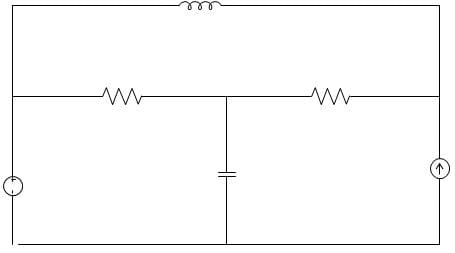Detailed Solution for Test: Series-Series Connection of Two Port Network - Question 7

Given that, b = 6, n = 3
Number of Links is given by, b – n + 1
= 6 – 3 + 1 = 4.

Test: Series-Series Connection of Two Port Network - Question 8

In the circuit given below, the maximum power that can be transferred to the resistor RL is _____________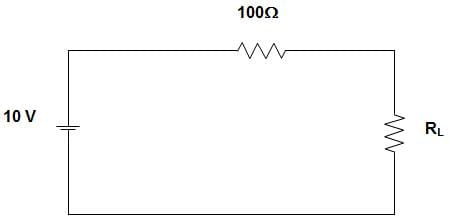Detailed Solution for Test: Series-Series Connection of Two Port Network - Question 8

For maximum power transfer to the load resistor RL, RL must be equal to 100 Ω.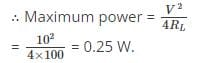Test: Series-Series Connection of Two Port Network - Question 9

In the circuit given below, the equivalent inductance is ____________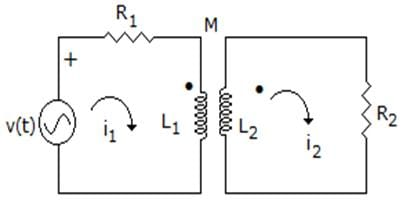Detailed Solution for Test: Series-Series Connection of Two Port Network - Question 9

Since, in one inductor current is leaving to dot and in other inductor current is entering to dot.
So, LEQ = L1 + L2 – 2M.

Test: Series-Series Connection of Two Port Network - Question 10

In the circuit given below, the number of node and branches are ______________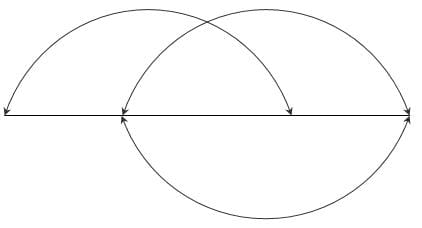Detailed Solution for Test: Series-Series Connection of Two Port Network - Question 10

In the given graph, there are 4 nodes and 6 branches.
Twig = n – 1 = 4 – 1 = 3
Link = b – n + 1 = 6 – 4 + 1 = 3.

Test: Series-Series Connection of Two Port Network - Question 11

In the circuit given below, the equivalent capacitance is ______________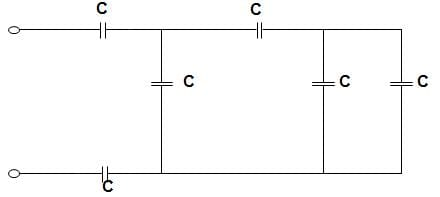Detailed Solution for Test: Series-Series Connection of Two Port Network - Question 11

The equivalent capacitance by applying the concept of series-parallel combination of the capacitance is,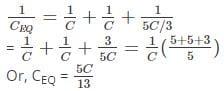123 c Energy delivered during talk time
E = ∫V(t)I(t) dt
Given, I (t) = 2 A = constant = 2 ∫ V(t)dt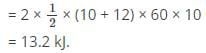Test: Series-Series Connection of Two Port Network - Question 12

The relation AD – BC = 1, (where A, B, C and D are the elements of a transmission matrix of a network) is valid for ___________

Detailed Solution for Test: Series-Series Connection of Two Port Network - Question 12

AD – BC = 1, is the condition for reciprocity for ABCD parameters, which shows that the relation is valid for reciprocal network. The ABCD parameters are obtained for the network which consists of resistance, capacitance and inductance, which indicates that it is a passive network.

Test: Series-Series Connection of Two Port Network - Question 13

Two networks are connected in series parallel connection. Then, the forward short-circuit current gain of the network is ____________

Detailed Solution for Test: Series-Series Connection of Two Port Network - Question 13

The forward short circuit current gain is given by,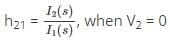So, when the two networks are connected in series parallel combination,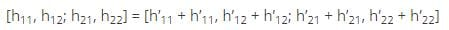So, h21 of total network will be sum of h parameter matrices.

Test: Series-Series Connection of Two Port Network - Question 14

In the circuit given below, the current through the 2 kΩ resistance is _____________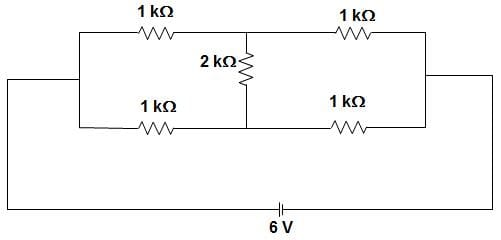Detailed Solution for Test: Series-Series Connection of Two Port Network - Question 14

We know that when a Wheatstone bridge is balanced, no current will flow through the middle resistance.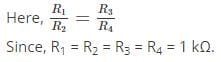Test: Series-Series Connection of Two Port Network - Question 15

In the circuit given below, the value of R is ___________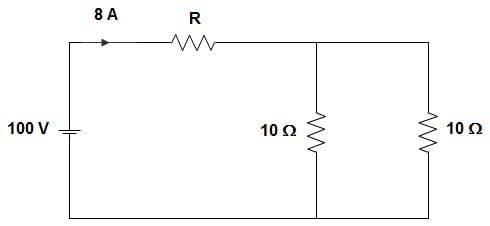Detailed Solution for Test: Series-Series Connection of Two Port Network - Question 15

The resultant R when viewed from voltage source = 100/8 = 12.5
∴ R = 12.5 – 10 || 10 = 12.5 – 5 = 7.55 Ω.

## Network Theory (Electric Circuits)

23 videos|63 docs|60 tests
 Use Code STAYHOME200 and get INR 200 additional OFF Use Coupon Code
Information about Test: Series-Series Connection of Two Port Network Page
In this test you can find the Exam questions for Test: Series-Series Connection of Two Port Network solved & explained in the simplest way possible. Besides giving Questions and answers for Test: Series-Series Connection of Two Port Network, EduRev gives you an ample number of Online tests for practice

## Network Theory (Electric Circuits)

23 videos|63 docs|60 tests